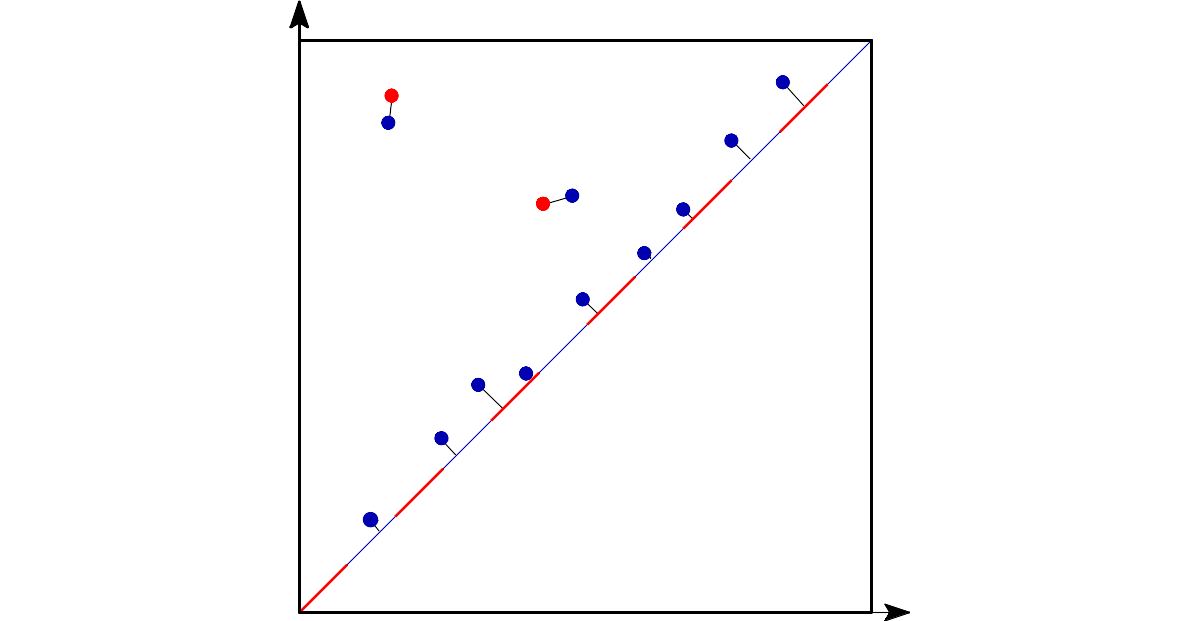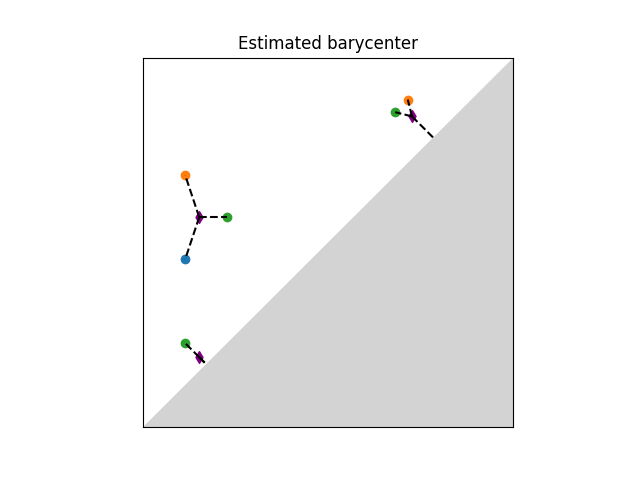# Wasserstein distance user manual¶

## Definition¶The q-Wasserstein distance measures the similarity between two persistence diagrams using the sum of all edges lengths (instead of the maximum). It allows to define sophisticated objects such as barycenters of a family of persistence diagrams. Author Theo Lacombe, Marc Glisse Since GUDHI 3.1.0 License MIT, BSD-3-Clause

The q-Wasserstein distance is defined as the minimal value achieved by a perfect matching between the points of the two diagrams (+ all diagonal points), where the value of a matching is defined as the q-th root of the sum of all edge lengths to the power q. Edge lengths are measured in norm p, for $$1 \leq p \leq \infty$$.

## Distance Functions¶

### Optimal Transport¶

Requires

Python Optimal Transport (POT) $$\geq$$ 0.5.1

This first implementation uses the Python Optimal Transport library and is based on ideas from “Large Scale Computation of Means and Cluster for Persistence Diagrams via Optimal Transport” .

gudhi.wasserstein.wasserstein_distance(X, Y, matching=False, order=1.0, internal_p=inf, enable_autodiff=False)[source]
Parameters
• X – (n x 2) numpy.array encoding the (finite points of the) first diagram. Must not contain essential points (i.e. with infinite coordinate).

• Y – (m x 2) numpy.array encoding the second diagram.

• matching – if True, computes and returns the optimal matching between X and Y, encoded as a (n x 2) np.array […[i,j]…], meaning the i-th point in X is matched to the j-th point in Y, with the convention (-1) represents the diagonal.

• order – exponent for Wasserstein; Default value is 1.

• internal_p – Ground metric on the (upper-half) plane (i.e. norm L^p in R^2); Default value is np.inf.

• enable_autodiff (bool) –

If X and Y are torch.tensor or tensorflow.Tensor, make the computation transparent to automatic differentiation. This requires the package EagerPy and is currently incompatible with matching=True.

Note

This considers the function defined on the coordinates of the off-diagonal points of X and Y and lets the various frameworks compute its gradient. It never pulls new points from the diagonal.

Returns

the Wasserstein distance of order q (1 <= q < infinity) between persistence diagrams with respect to the internal_p-norm as ground metric. If matching is set to True, also returns the optimal matching between X and Y.

### Hera¶

This other implementation comes from Hera (BSD-3-Clause) which is based on “Geometry Helps to Compare Persistence Diagrams”  by Michael Kerber, Dmitriy Morozov, and Arnur Nigmetov.

gudhi.hera.wasserstein_distance(X: numpy.ndarray[float64], Y: numpy.ndarray[float64], order: float = 1, internal_p: float = inf, delta: float = 0.01) → float

Compute the Wasserstein distance between two diagrams. Points at infinity are supported.

Parameters
• X (n x 2 numpy array) – First diagram

• Y (n x 2 numpy array) – Second diagram

• order (float) – Wasserstein exponent W_q

• internal_p (float) – Internal Minkowski norm L^p in R^2

• delta (float) – Relative error 1+delta

Returns

Approximate Wasserstein distance W_q(X,Y)

Return type

float

### Basic example¶

This example computes the 1-Wasserstein distance from 2 persistence diagrams with Euclidean ground metric. Note that persistence diagrams must be submitted as (n x 2) numpy arrays and must not contain inf values.

import gudhi.wasserstein
import numpy as np

dgm1 = np.array([[2.7, 3.7],[9.6, 14.],[34.2, 34.974]])
dgm2 = np.array([[2.8, 4.45],[9.5, 14.1]])

message = "Wasserstein distance value = " + '%.2f' % gudhi.wasserstein.wasserstein_distance(dgm1, dgm2, order=1., internal_p=2.)
print(message)


The output is:

Wasserstein distance value = 1.45


We can also have access to the optimal matching by letting matching=True. It is encoded as a list of indices (i,j), meaning that the i-th point in X is mapped to the j-th point in Y. An index of -1 represents the diagonal.

import gudhi.wasserstein
import numpy as np

dgm1 = np.array([[2.7, 3.7],[9.6, 14.],[34.2, 34.974]])
dgm2 = np.array([[2.8, 4.45], [5, 6], [9.5, 14.1]])
cost, matchings = gudhi.wasserstein.wasserstein_distance(dgm1, dgm2, matching=True, order=1, internal_p=2)

message_cost = "Wasserstein distance value = %.2f" %cost
print(message_cost)
dgm1_to_diagonal = matchings[matchings[:,1] == -1, 0]
dgm2_to_diagonal = matchings[matchings[:,0] == -1, 1]
off_diagonal_match = np.delete(matchings, np.where(matchings == -1), axis=0)

for i,j in off_diagonal_match:
print("point %s in dgm1 is matched to point %s in dgm2" %(i,j))
for i in dgm1_to_diagonal:
print("point %s in dgm1 is matched to the diagonal" %i)
for j in dgm2_to_diagonal:
print("point %s in dgm2 is matched to the diagonal" %j)


The output is:

Wasserstein distance value = 2.15
point 0 in dgm1 is matched to point 0 in dgm2
point 1 in dgm1 is matched to point 2 in dgm2
point 2 in dgm1 is matched to the diagonal
point 1 in dgm2 is matched to the diagonal


## Barycenters¶

Requires

Python Optimal Transport (POT) $$\geq$$ 0.5.1

A Frechet mean (or barycenter) is a generalization of the arithmetic mean in a non linear space such as the one of persistence diagrams. Given a set of persistence diagrams $$\mu_1 \dots \mu_n$$, it is defined as a minimizer of the variance functional, that is of $$\mu \mapsto \sum_{i=1}^n d_2(\mu,\mu_i)^2$$. where $$d_2$$ denotes the Wasserstein-2 distance between persistence diagrams. It is known to exist and is generically unique. However, an exact computation is in general untractable. Current implementation available is based on (Turner et al., 2014),  and uses an EM-scheme to provide a local minimum of the variance functional (somewhat similar to the Lloyd algorithm to estimate a solution to the k-means problem). The local minimum returned depends on the initialization of the barycenter. The combinatorial structure of the algorithm limits its performances on large scale problems (thousands of diagrams and of points per diagram).Illustration of Frechet mean between persistence diagrams.

gudhi.wasserstein.barycenter.lagrangian_barycenter(pdiagset, init=None, verbose=False)[source]
Parameters
• pdiagset – a list of numpy.array of shape (n x 2) (n can variate), encoding a set of persistence diagrams with only finite coordinates.

• init (int, or (n x 2) np.array) – The initial value for barycenter estimate. If None, init is made on a random diagram from the dataset. Otherwise, it can be an int (then initialization is made on pdiagset[init]) or a (n x 2) numpy.array enconding a persistence diagram with n points.

• verbose (boolean) – if True, returns additional information about the barycenter.

Returns

If not verbose (default), a numpy.array encoding the barycenter estimate of pdiagset (local minimum of the energy function). If pdiagset is empty, returns None. If verbose, returns a couple (Y, log) where Y is the barycenter estimate, and log is a dict that contains additional informations:

• ”groupings”, a list of list of pairs (i,j). Namely, G[k] = [...(i, j)...], where (i,j) indicates that pdiagset[k][i] is matched to Y[j] if i = -1 or j = -1, it means they represent the diagonal.

• ”energy”, float representing the Frechet energy value obtained. It is the mean of squared distances of observations to the output.

• ”nb_iter”, int number of iterations performed before convergence of the algorithm.

### Basic example¶

This example estimates the Frechet mean (aka Wasserstein barycenter) between four persistence diagrams. It is initialized on the 4th diagram. As the algorithm is not convex, its output depends on the initialization and is only a local minimum of the objective function. Initialization can be either given as an integer (in which case the i-th diagram of the list is used as initial estimate) or as a diagram. If None, it will randomly select one of the diagrams of the list as initial estimate. Note that persistence diagrams must be submitted as (n x 2) numpy arrays and must not contain inf values.

from gudhi.wasserstein.barycenter import lagrangian_barycenter
import numpy as np

dg1 = np.array([[0.2, 0.5]])
dg2 = np.array([[0.2, 0.7]])
dg3 = np.array([[0.3, 0.6], [0.7, 0.8], [0.2, 0.3]])
dg4 = np.array([])
pdiagset = [dg1, dg2, dg3, dg4]
bary = lagrangian_barycenter(pdiagset=pdiagset,init=3)

message = "Wasserstein barycenter estimated:"
print(message)
print(bary)


The output is:

Wasserstein barycenter estimated:
[[0.27916667 0.55416667]
[0.7375     0.7625    ]
[0.2375     0.2625    ]]
`

### Tutorial¶

This notebook presents the concept of barycenter, or Fréchet mean, of a family of persistence diagrams.